Next: 4.1.1.1 Contributions to Up: 4.1 Contributions to the Previous: 4.1 Contributions to the

## 4.1.1 The Basic Model

For MESFETs and HEMTs the well known intrinsic eight-element small-signal equivalent circuit is shown in Fig. 4.1, as published in . The extraction of parasitic elements from measurements is performed according to standard procedures [36,230] which are described below. Contrary to the classical separation of the intrinsic device and the parasitic environment, Fig. 4.1 additionally shows a simulator shell, which includes the intrinsic shell and the parasitic resistances. This is useful as the semiconductor contributions to the resistances are a genuine part of the simulation domain. However, parasitic capacitances and inductances can better be obtained from gate width dependent measurements, so that they are considered extrinsic for the physical simulation. Extended equivalent circuits were suggested, e.g. by   to include leakage and impact ionization phenomena in InAlAs/InGaAs based HEMTs.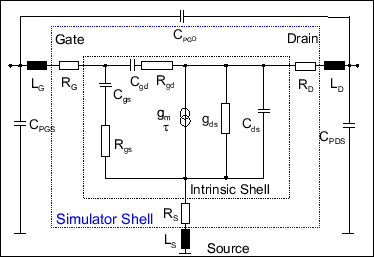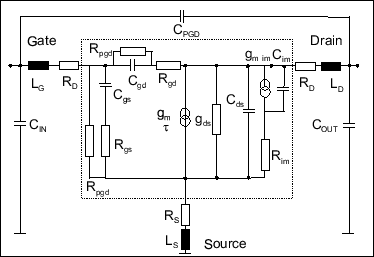Fig. 4.2 shows an extended equivalent circuit for a mm-wave HEMT. In the input circuit two resistances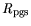and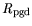are added to account for the gate leakage. In the output circuit three additional elements are added: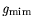introduces a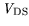dependent contribution to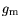due to impact ionization and the combination of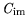and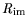present an additional time constant explained below. Genetic algorithms were suggested in  to determine small-signal equivalent elements, and are also used in this work. This circuit, usually applied for noise modeling, is used to extract breakdown information from measured S-parameters at highbias in combination with standard procedures. In a one-dimensional linear charge control model, as given in , the transconductanceis described as: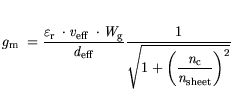(4.1)

where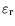is the relative dielectric constant,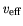the effective carrier velocity,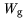the gate width, and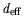an effective gate-to-channel separation.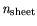is the sheet charge density and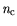a reference sheet concentration defined as:(4.2)

with:is a critical field for the onset of a "saturated" behavior andis an effective channel capacity assuming a fixed distance between channel charge and gate in a simple capacitor model.

As can be seen from (4.1), both a relatively higher sheet densityand a higher effective carriervelocity lead to higher. This explains the differences of the material systems AlGaAs/InGaAs and InAlAs/InGaAs. As an example, InAlAs/InGaAs transistors have both a higher sheet density and higher effective carrier velocities than AlGaAs/InGaAs HEMTs. Furthermore, the difference of DC- and RF-can be explained due carrier generation/recombination resulting in changes of. Neither velocity saturation nor parasitic charge modulation are included in this model. Describing velocity saturation a term called modulation efficiency (ME) can be derived writing an intrinsic delay time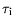as: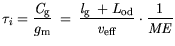(4.3)

with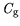the total gate-charge. Adding to the purely one-dimensional approach of (4.3), the effects of an additionalbias, i.e., a the two-dimensional consideration, are included using an extension of the symmetric space charge region by a length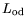. This length describes the drain side extension of the space charge region. Parasitic charge modulation is further included by setting an upper limit towards the modulation efficiency ME. The model assumes a saturated effective carrier velocity, with no changes as a function of. Thedependence of the carrier velocities is to be understood by two-dimensional device simulation.

SubsectionsNext: 4.1.1.1 Contributions to Up: 4.1 Contributions to the Previous: 4.1 Contributions to the
Quay
2001-12-21